# 5 NUMA Support

## 5.1 NUMA Hardware

Figure 2.3是最简单的NUMA形式，处理器可以有自己的本地内存，访问本地内存和其他处理器的本地内存的开销区别不大，即NUMA factor比较低。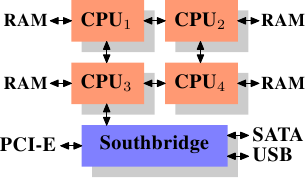Figure 2.3: Integrated Memory Controller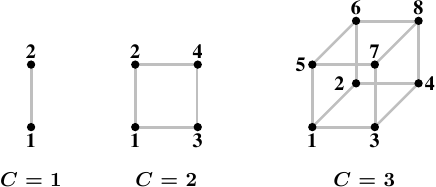Figure 5.1: Hypercubes

## 5.3 Published Information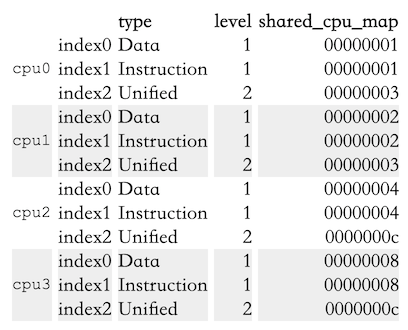Table 5.1: sysfs Information for Core 2 CPU Caches

• 每个核（为啥cpu0到cpu3都是核是从另一个地方知道的）拥有三个cache：L1d、L1i、L2
• L1d和L1i是每个核独占的，This is indicated by the bitmap in shared_cpu_map having only one set bit.
• cpu0和cpu1共享L1、cpu2和cpu3共享L2。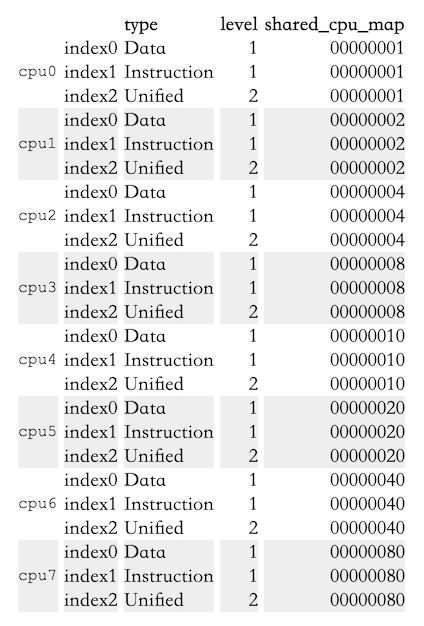Table 5.2: sysfs Information for Opteron CPU Caches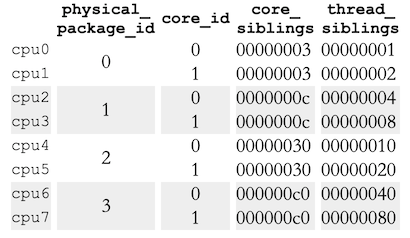Table 5.3: sysfs Information for Opteron CPU Topology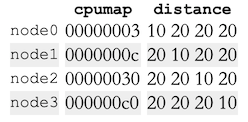Table 5.4: `sysfs` Information for Opteron Nodes

• 这个机器有4个处理器。
• 每个处理器自成一个node，可以从`cpumap`文件里的bit看出来。
• `distance`文件描述了访问每个node的开销。本地开销为10，远程开销都是20。（不过这里的信息并不准确，至少有一个处理器要连接到南桥，所以至少有一对处理器的开销比20大）
• 所有处理器构成一个二维超立方体（Figure 5.1）

## 5.4 Remote Access Costs

AMD文档里写了4插口机器的NUMA开销：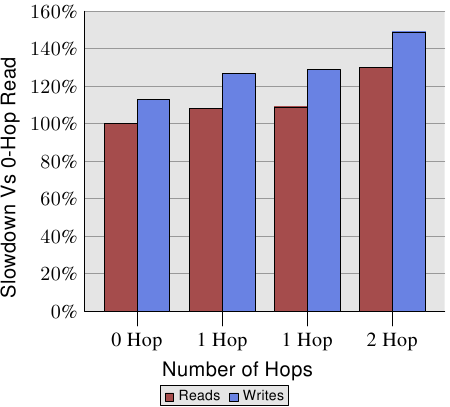Figure 5.3: Read/Write Performance with Multiple Nodes

``````00400000 default file=/bin/cat mapped=3 N3=3
00504000 default file=/bin/cat anon=1 dirty=1 mapped=2 N3=2
00506000 default heap anon=3 dirty=3 active=0 N3=3
38a9000000 default file=/lib64/ld-2.4.so mapped=22 mapmax=47 N1=22
38a9119000 default file=/lib64/ld-2.4.so anon=1 dirty=1 N3=1
38a911a000 default file=/lib64/ld-2.4.so anon=1 dirty=1 N3=1
38a9200000 default file=/lib64/libc-2.4.so mapped=53 mapmax=52 N1=51 N2=2
38a933f000 default file=/lib64/libc-2.4.so
38a943f000 default file=/lib64/libc-2.4.so anon=1 dirty=1 mapped=3 mapmax=32 N1=2 N3=1
38a9443000 default file=/lib64/libc-2.4.so anon=1 dirty=1 N3=1
38a9444000 default anon=4 dirty=4 active=0 N3=4
2b2bbcdce000 default anon=1 dirty=1 N3=1
2b2bbcde4000 default anon=2 dirty=2 N3=2
2b2bbcde6000 default file=/usr/lib/locale/locale-archive mapped=11 mapmax=8 N0=11
7fffedcc7000 default stack anon=2 dirty=2 N3=2
``````

Figure 5.2: Content of `/proc/PID/numa_maps`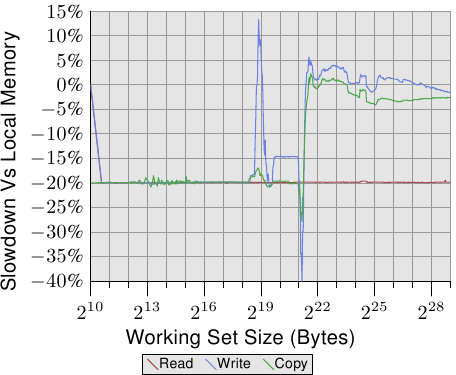Figure 5.4: Operating on Remote Memory